## Strategy Report

From the strategy details page, you can access a detailed report for each history type available. You can select start and end dates to filter the history and change the initial balance used in the calculations.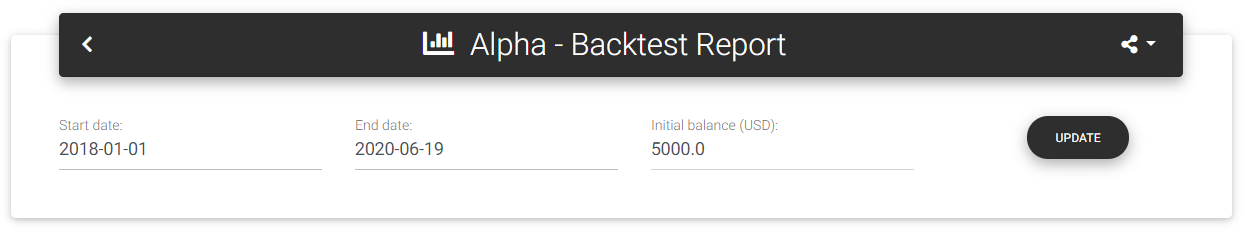Strategy report

### Main statistics

In the first part of the report, you will see the leading statistics and a chart with the equity curve. The card "Main statistics" shows the following information: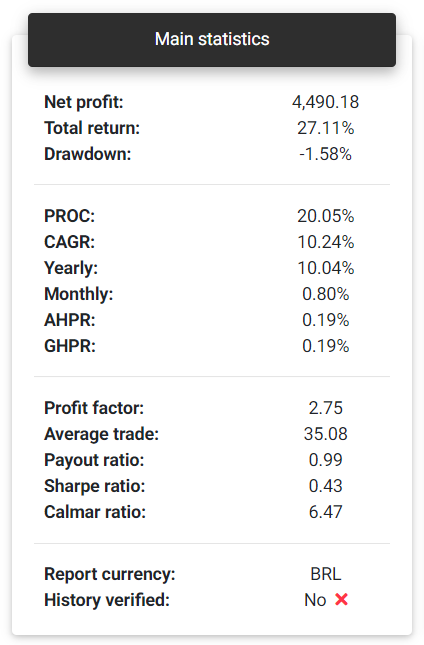Main statistics

Net profit: This is the total net profit achieved by the strategy in the selected period. This profit calculation considers the trading costs defined for the symbol, so it may be slightly different from what you see on the strategy's main page.

Total return: Indicates the strategy's percentage return, based on the net profit and initial balance.

Tip: All percentage returns are dependent on the initial balance defined for the strategy.

Drawdown: This is the maximum percentage drawdown verified in the balance. This measure indicates how much your balance has decreased after reaching any maximum point.

PROC: Pessimistic Return on Capital is an adjusted measure of percentage return, taking into account the strategy's win rate and average profit. This measure can give a more conservative indication of the expected return for a strategy.

CAGR: Compound Annual Growth Rate is the annual return rate needed to obtain the final balance from strategy's initial balance.

Yearly: Yearly return calculated for the strategy.

Monthly: Monthly return calculated for the strategy.

AHPR: Average Holding Period Return is the average return of each trade, which is the average balance change for each trade.

GHPR: Geometric Holding Period Return is similar to AHPR but applies a weight with the number of trades.

Profit factor: The profit factor is calculated as the ratio between the sum of all profits and all losses.

Average trade: This is the average expected result for each trade or the average profit obtained from the trades.

Payout ratio: It's the ratio between the average profit and the average loss. It gives an insight into the strategy's risk-reward ratio. Values less than 1.0 indicate that the risk of loss for each operation is much greater than the probability of gain.

Sharpe ratio: It's the ratio of strategy's net return compared to a risk-free rate and the standard deviation of these returns. To simplify the calculations, in this case, the risk-free rate is considered zero.

Calmar ratio: This metric can also be called the Drawdown ratio. It's the ratio between the average annual return and the maximum drawdown of the strategy.

Report currency: Shows the currency used in the report, obtained from the history. The currency used will be the one that is most frequent in history.

History verified: Indicates whether historical data was sent from a publishing agent directly from trading account or not. Data uploaded manually using files is not considered verified, since it can be manipulated easily.

Notice: We have taken some security measures to ensure publishing agents' messages authentication to prevent fake data. However, systems and messages on the Internet are susceptible to attacks, and there is no full guarantee that the data came from a reliable source. Therefore, this verified history information cannot be considered absolute truth.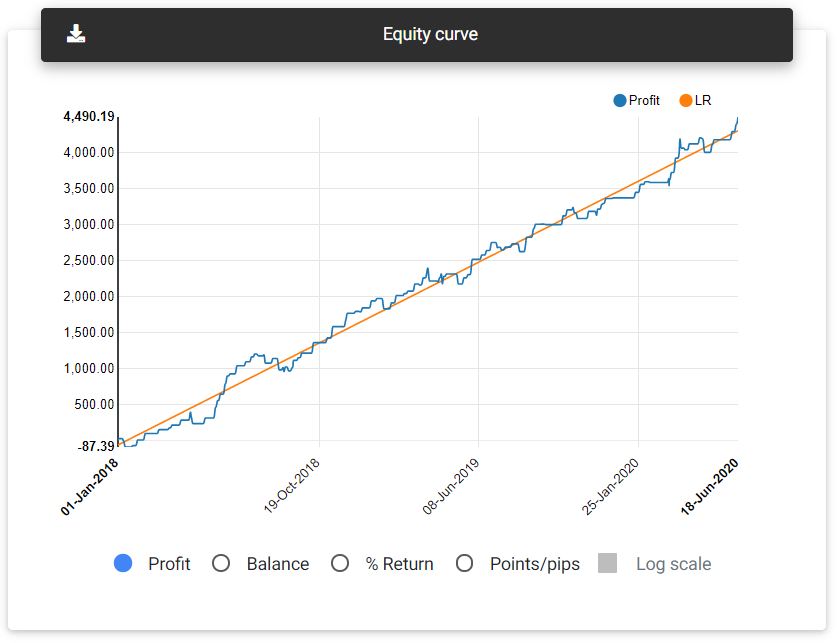Equity curve

### Return analysis

##### Monthly returns

This card shows a bar chart with the strategy returns for each month available in history. You can see the results in percentage return, financial values, or points/pips. It's possible to select a specific year at the bottom of the chart to check the results.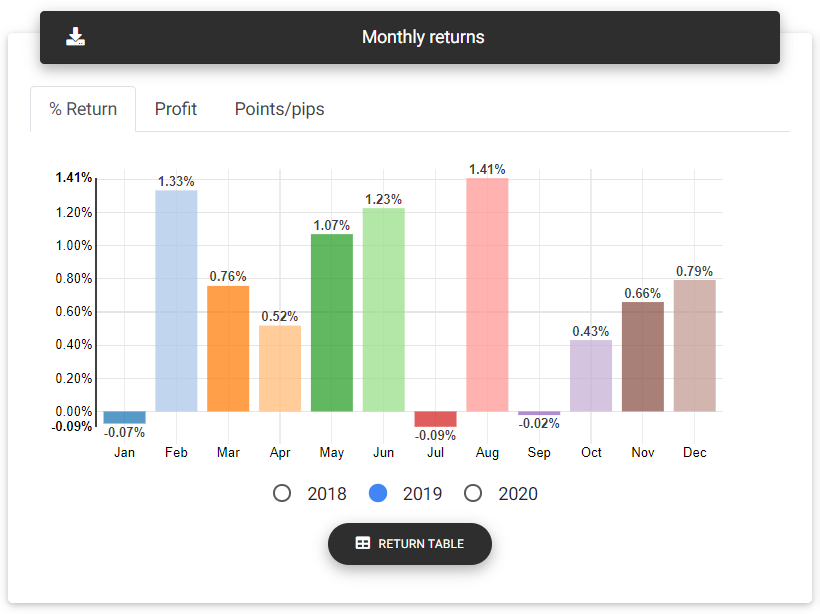Monthly returns

##### Yearly returns

This card shows the accumulated result in each year in which the trades took place. You can see the results in percentage return, financial values, or points/pips.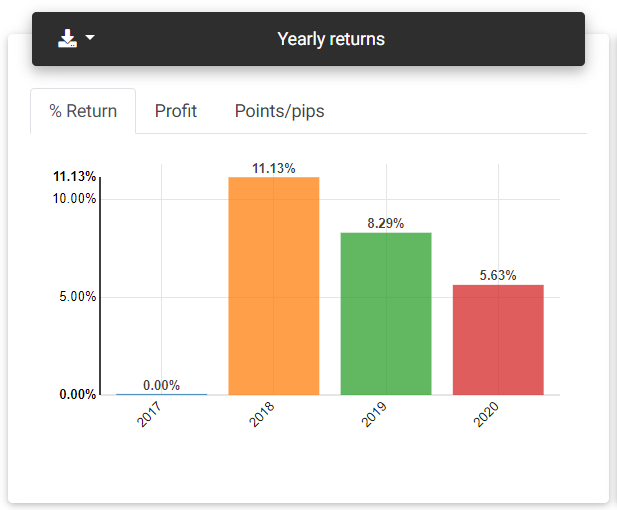Yearly returns

##### Latest returns

From the charts presented in this card, you can see the strategy's recent results, referring to the day, week, and month, compared with the previous period.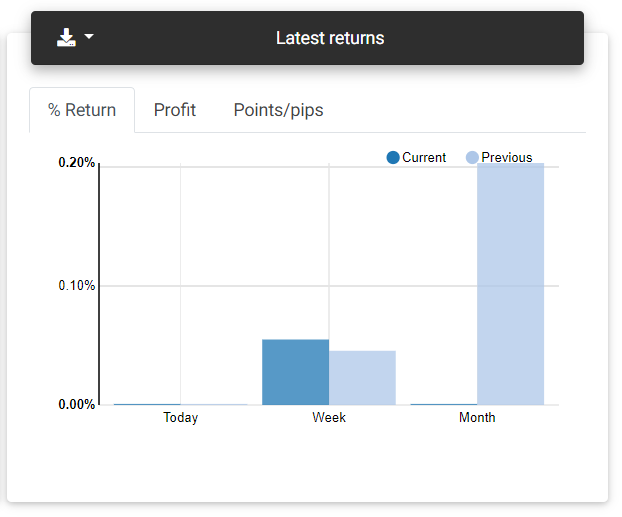Latest returns

##### Daily returns

On this card, you find a table with the returns obtained each day, ending on the last day there was a trade on the history.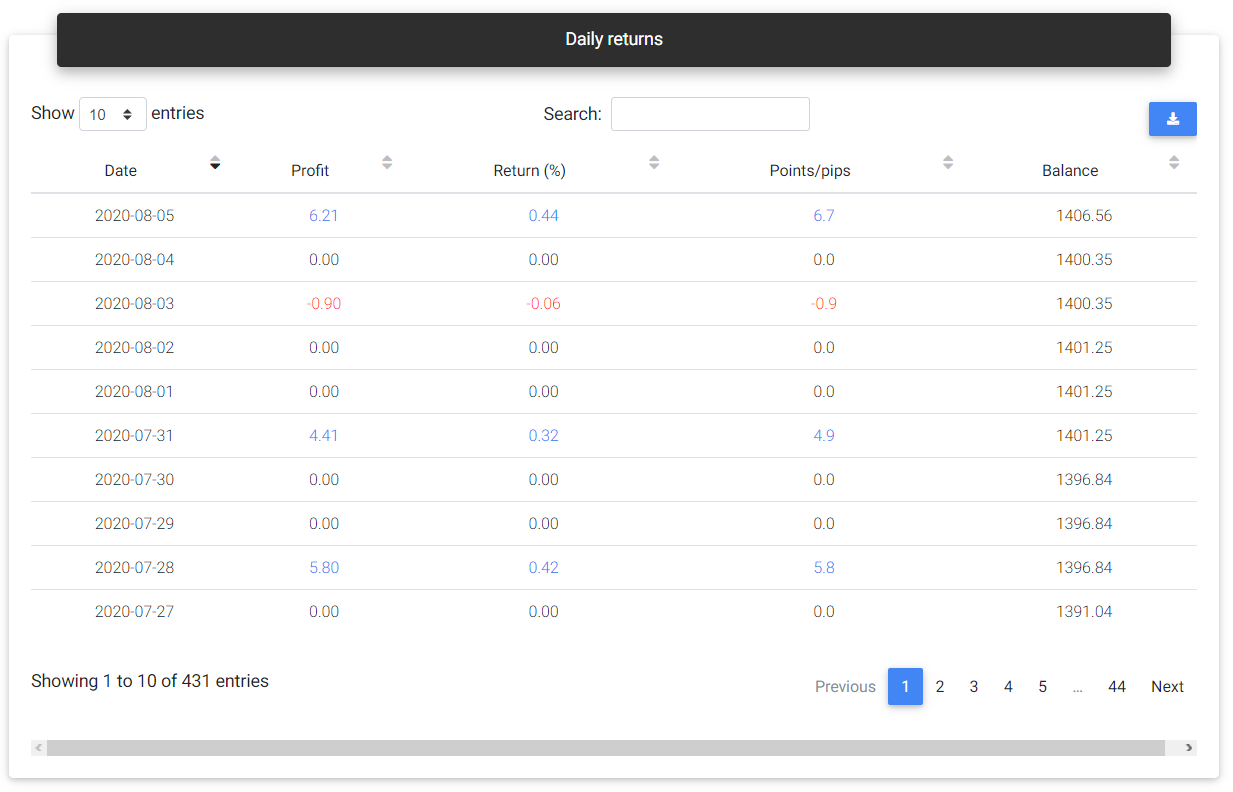Daily returns

##### Return statistics

Today: Percentage return obtained on the current day.

This week: Percentage return achieved in the current week.

This month: Percentage return achieved in the current month.

This year: Percentage return achieved in the current year.

Best year: Highest annual percentage return ever achieved by the strategy.

Worst year: Lowest annual percentage return ever achieved by the strategy.

Best month: Highest monthly percentage return ever achieved by the strategy.

Worst month: Lowest monthly percentage return ever achieved by the strategy.

Monthly Sharpe: This is the Sharpe ratio calculated monthly.

### Drawdown and risk

##### Risk of ruin

The risk of ruin considers past results to calculate the probability of losing some part or even the total capital invested in the strategy. Ideally, the strategy should have a higher probability of losing smaller portions of the capital and reducing probability as a greater loss is simulated. With a chart's aid, we expect to see a curve with a decline in the risk of ruin to something near zero as the loss is close to the entire capital.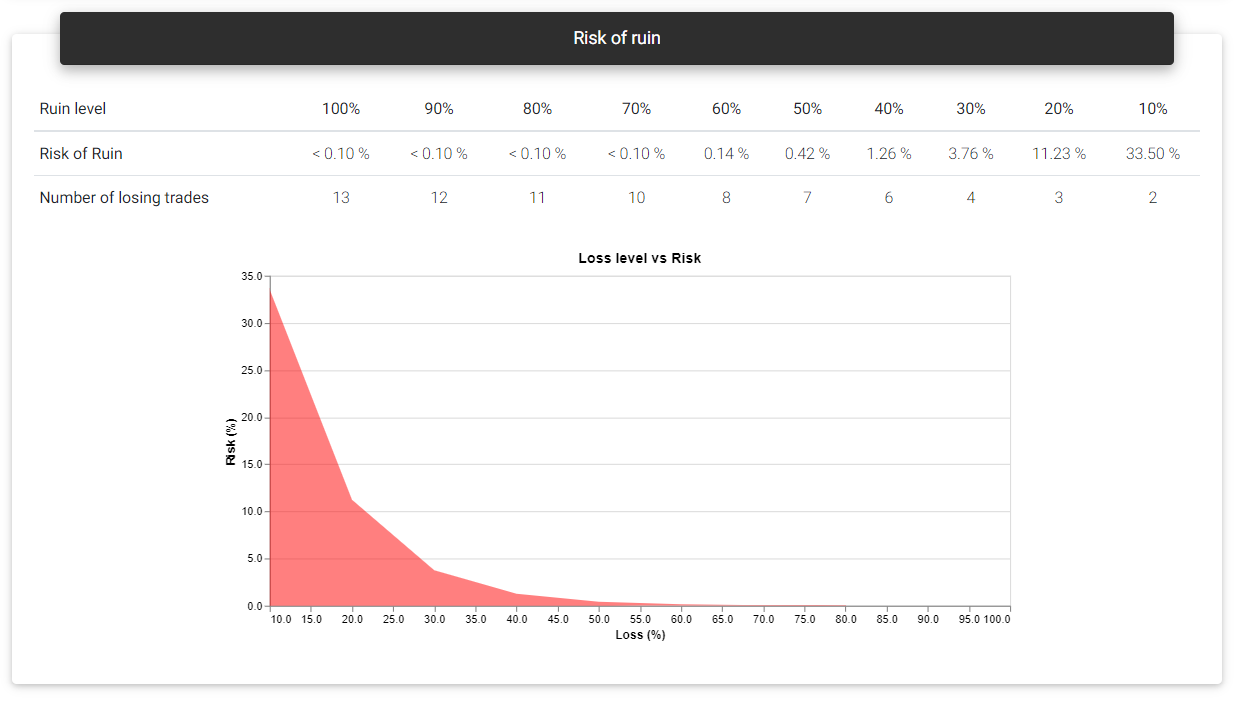Risk of ruin

##### Drawdown statistics

Maximum relative: This is the highest percentage drawdown verified in history. The percentage drawdown tends to be higher in the first trades if the history is based on fixed lot trades. In brackets, you see the financial value corresponding to this drawdown.

Maximum absolute: Largest financial drawdown value verified. In brackets, it's shown the percentage change corresponding to this drawdown.

From initial balance: The largest financial drawdown is applied to the initial balance to give you an idea of how much this value represents in percentage terms if you faced the drawdown in the first trades.

Maximum duration: Shows the length of the longest drawdown measured in days.

Average duration: This is the average length of a drawdown measured in days.

Recovery factor: This is the total net profit divided by the maximum drawdown verified. This number gives an idea of the strategy's recovering power from drawdown periods.

##### Longest drawdowns

Drawdowns are common to all strategies. Strategies spend most of their time on some drawdown, as any negative trade will start or continue such a move. What you should note is the duration and size of these drawdowns, which can represent a considerable loss of capital for a long time. For example, if a strategy takes a year to recover from a drawdown, it may not worth putting it into a real account. On this card, a chart gives you a general idea of ​​the drawdown periods faced by the strategy. The primary curve is profit growth, with the five most extensive drawdown periods indicated in different colors. Also, below the profit chart, three curves representing the same drawdown levels are plotted, one in points (volume independent), another with financial value, and the percentage drawdown. These curves will appear in red whenever the strategy continues in a drawdown period, and by hovering them, you can see the corresponding values.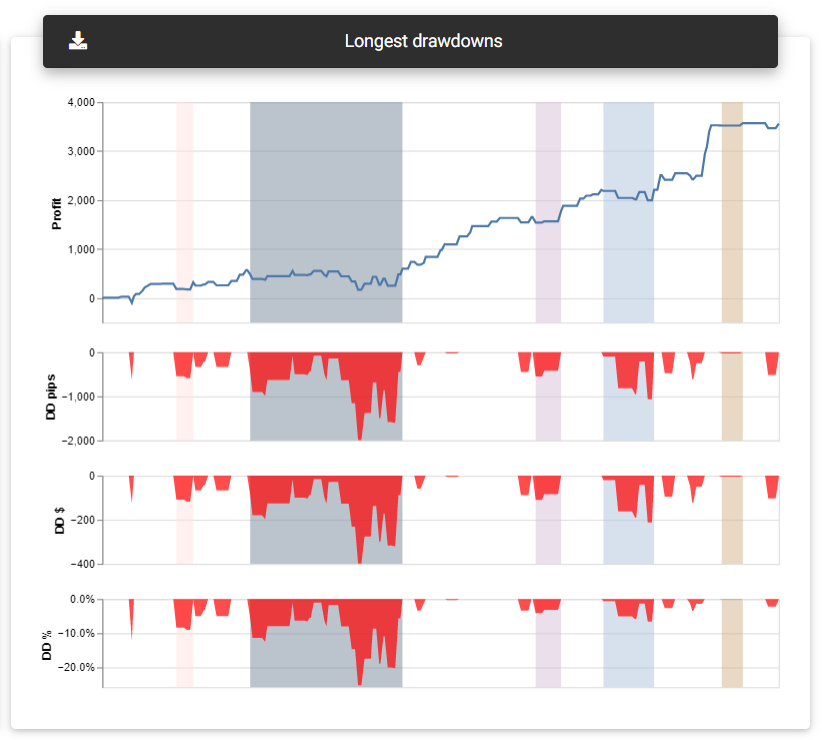Longest drawdowns

##### Drawdowns details

This table will show all the strategy's drawdown periods, indicating the start and end dates, duration, and corresponding values in percentage, financial, and points/pips.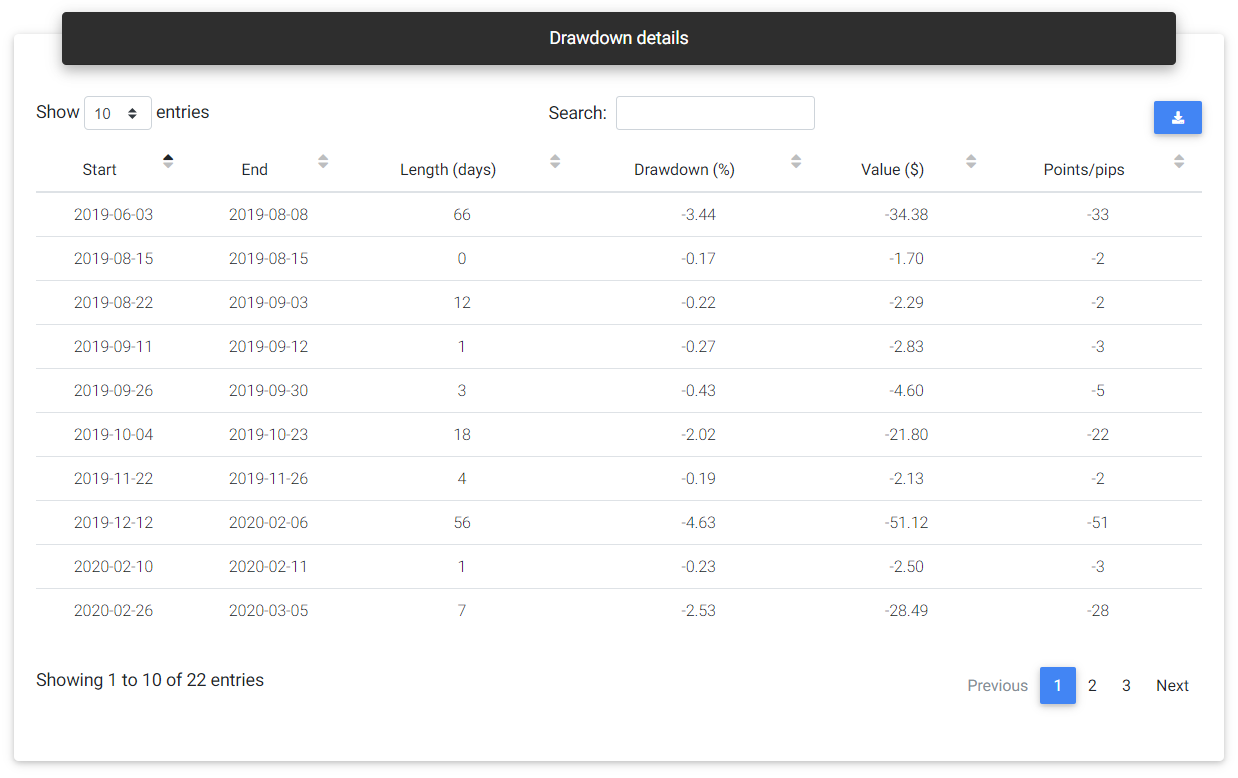Drawdown details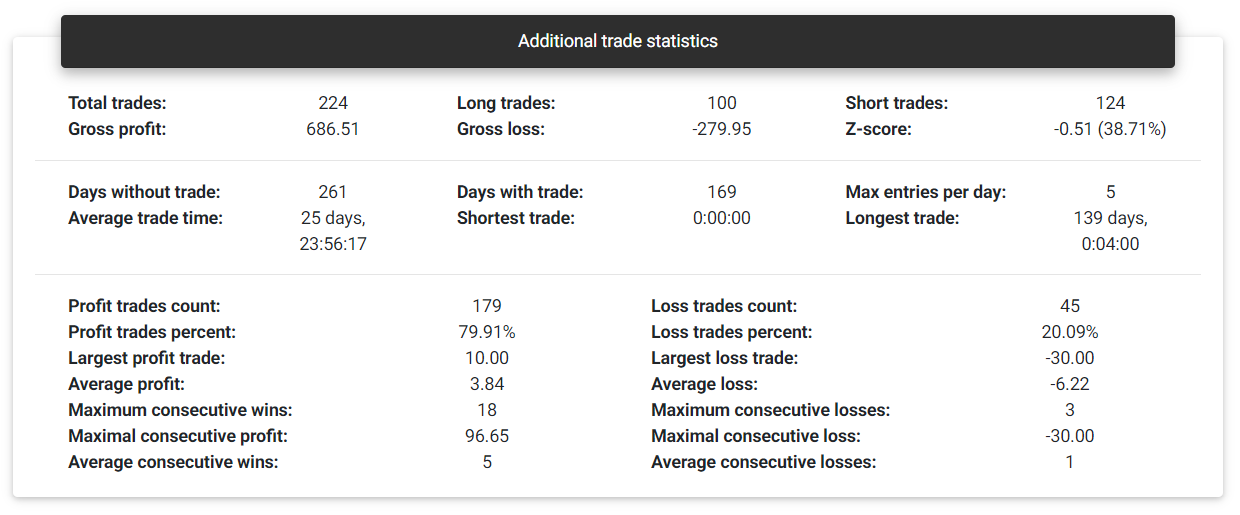Total trades: This is the total number of trades observed in history. The app counts each closed transaction as an individual trade. If there are partial closes, each one will be considered a different trade.

Gross profit: This is the sum of all trades that ended with a profit.

Gross loss: This is the sum of all trades that ended with a loss.

Z-score: With the Z-score, it is possible to assess whether the strategy will likely present sequences of gains or losses or whether the results will alternate. Negative Z-score values from -2.0 indicate a 95% probability that another profitable trade will follow a profitable trade, and likewise, a loss will be followed by another loss. Positive values starting from 2.0 suggest that with a 95% probability, a losing trade will follow a profitable trade and vice versa. Z-score values between -2.0 and +2.0 don't lead to a conclusion regarding the dependency between trades.

Days without trade: Number of days that there was no trade with this strategy.

Days with trade: Number of days that there was trade with this strategy.

Max entries per day: Maximum number of trades in a single day.

Profit trades count: Number of trades that ended with positive or zero results.

Profit trades percent: Percentage trades that ended with positive or zero results.

Average profit: Average result from trades with profit.

Average loss: Average result from trades with loss.

Maximum consecutive wins: Maximum number of consecutive trades with profit.

Maximum consecutive losses: Maximum number of consecutive trades with loss.

Maximal consecutive profit: Highest profit achieved in consecutive trades.

Maximal consecutive loss: Highest loss achieved in consecutive trades.

Average consecutive wins: Average number of consecutive profitable trades.

Average consecutive losses: Average number of consecutive losing trades.

This card presents a chart with each trade result, with the profit represented on the y-axis and the x-axis trade duration in minutes.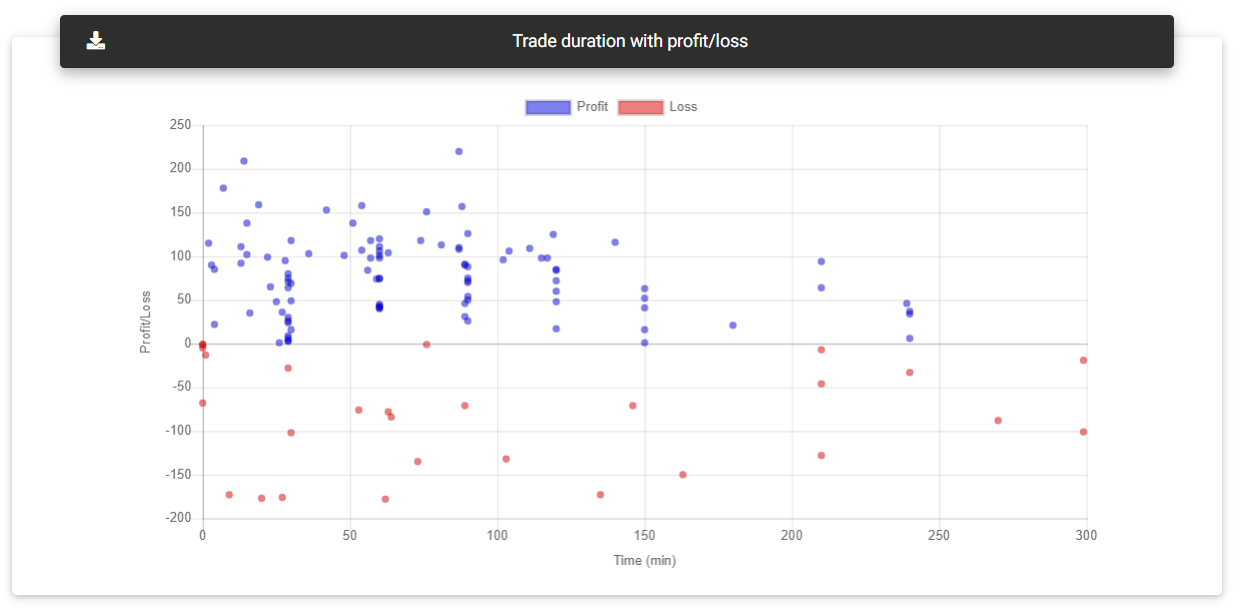##### Entries charts

Entries charts show the number of trades started for each hour of the day, day of the week, and month.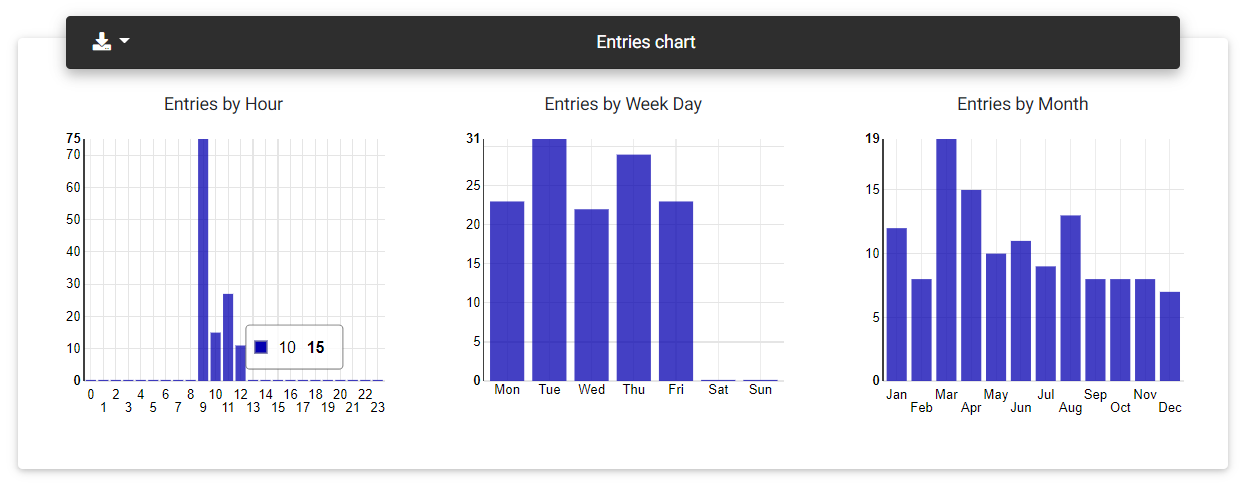Entries charts

##### Profit/Loss charts

These charts show the sum of profits and losses obtained at each hour of the day, day of the week and month.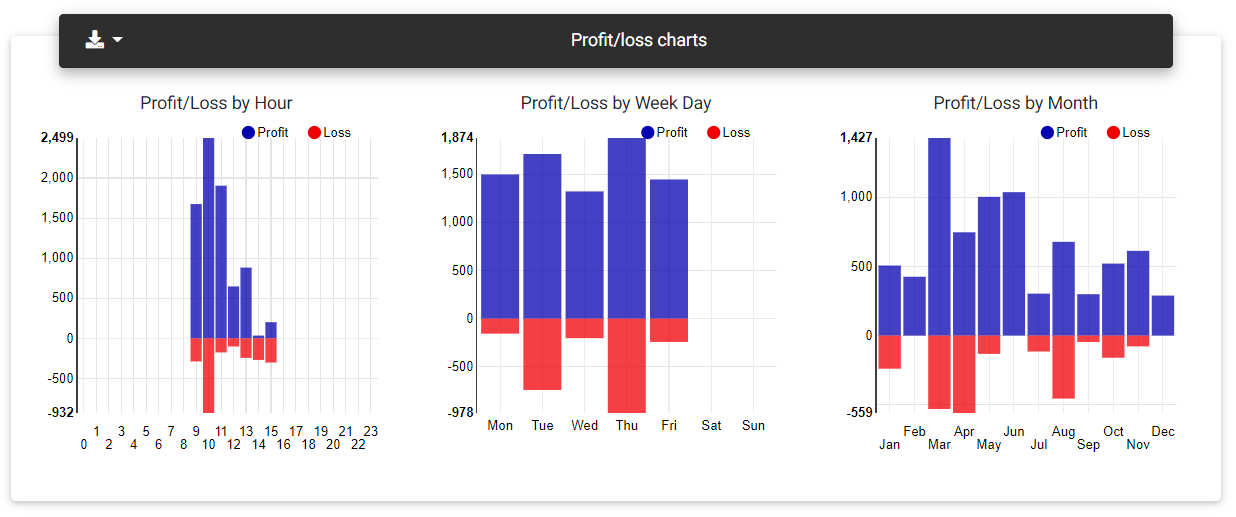Profit/loss charts

##### MFE/MAE with profit

MFE is the Maximum Favorable Excursion achieved during the trade, indicating the largest price swing favorable to the trade, and consequently, the highest open profit. MAE (Maximum Adverse Excursion) is the opposite, meaning the largest price swing against the trade, representing the largest open loss. This chart shows a plot that relates the measures of MFE and MAE with the final trade result.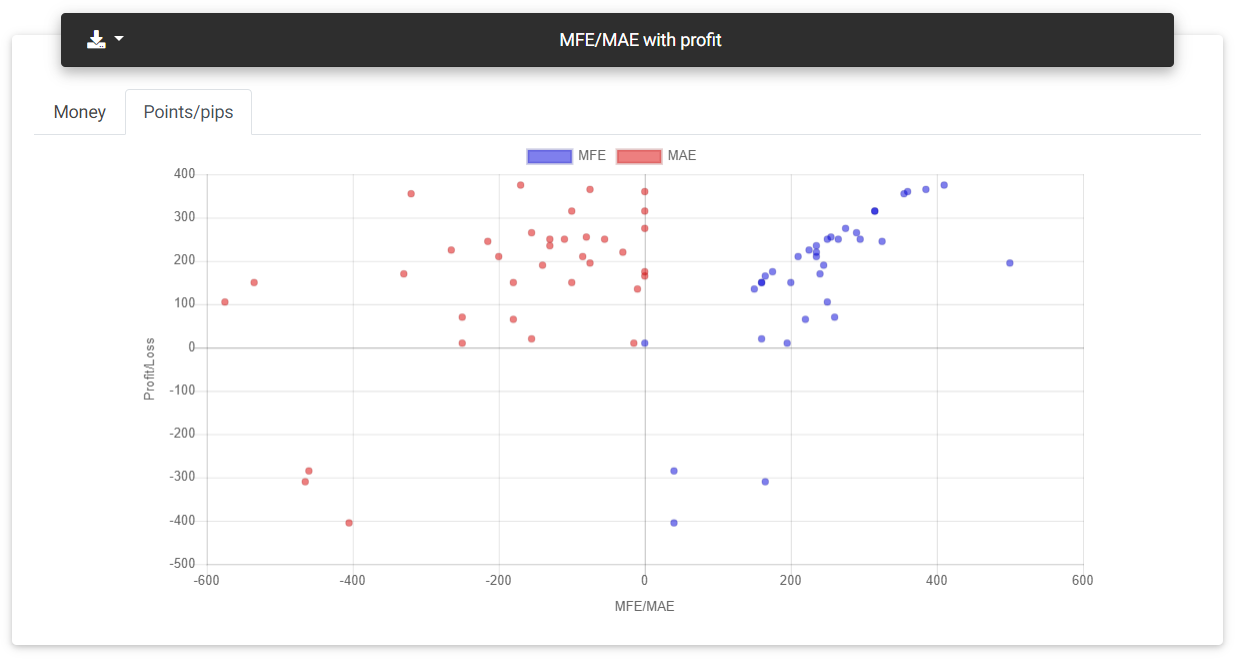MAE/MFE

### Costs, benchmark, and forecast

##### Costs and slippage impact

If the strategy's symbol has cost information configured, this chart will compare the equity without costs and considering trading costs. It is possible to simulate other trading costs and slippage values to get an idea of their impact on the strategy's result. In this chart, it's possible to see that even a strategy with positive backtest results could not be tradeable if high trading costs are applied.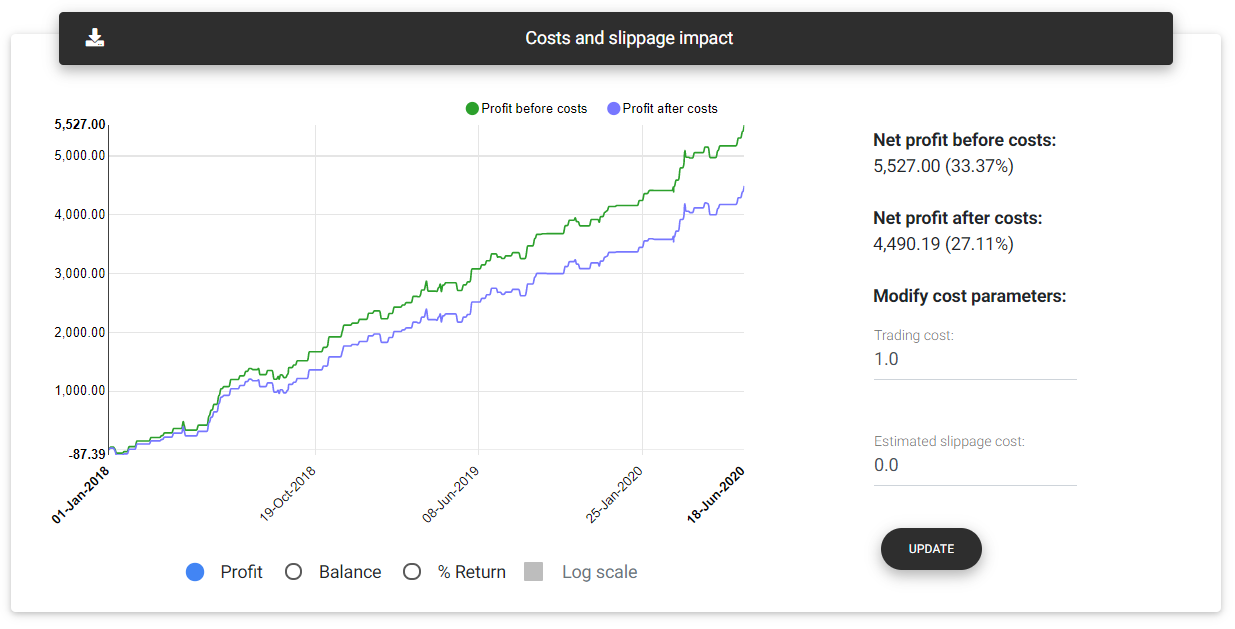Costs and slippage

##### Benchmark

On this card, you can compare your strategy's performance with the benchmark symbol chosen, and it's also possible to select other benchmarks for evaluation directly in the report. The app normalizes the opening balance for the two histories, and the percentage returns obtained by the benchmark and strategy itself are applied. Low initial balances tend to overestimate the strategy's results. Always try to choose an initial deposit that represents the financial volume proportional to the traded instrument.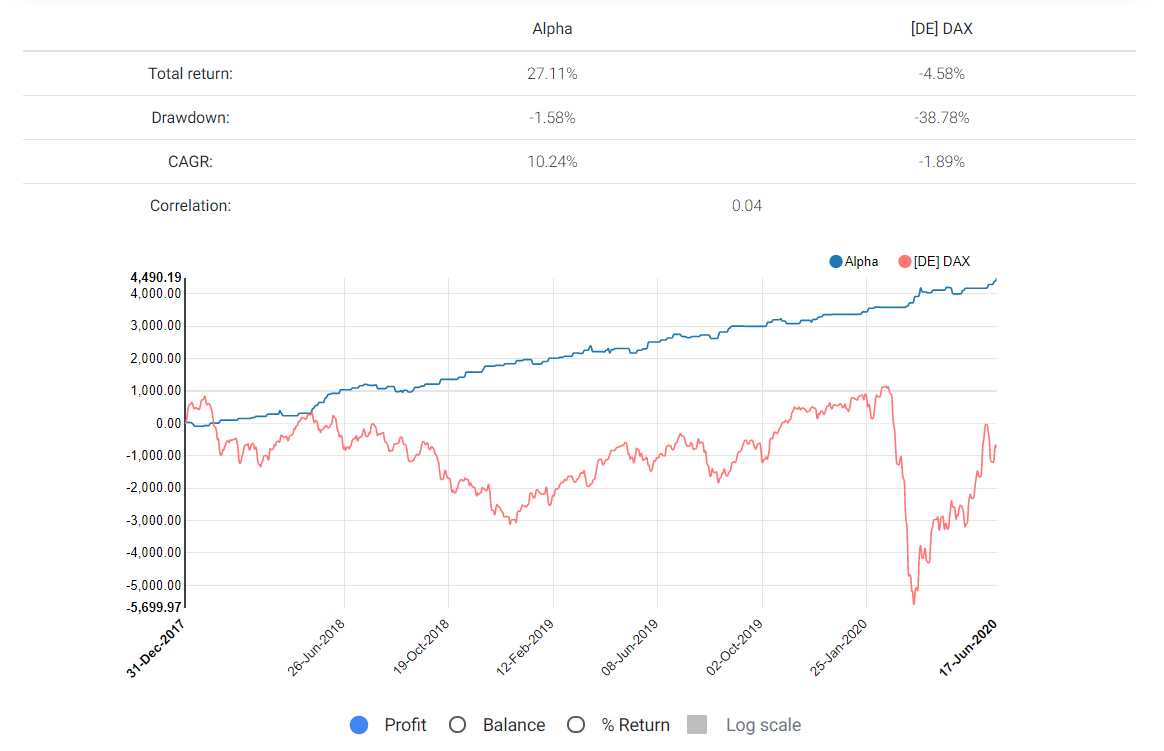Benchmark

##### Forecast

Based on backtest history, the app generates a prediction model that will give a possible direction to the strategy's balance in the following six months. If the report is built with backtest data, only the forecast will be shown, with the bands indicating the confidence levels or margin of error. If the data comes from a demo or real account, the app will attempt to create a model from the backtest until live trading started. Then the results from demo or real accounts will be presented as OOS (out of sample) and overlaid on the model's prediction. With that, it is possible to assess whether the results from live trades deviate from the expected.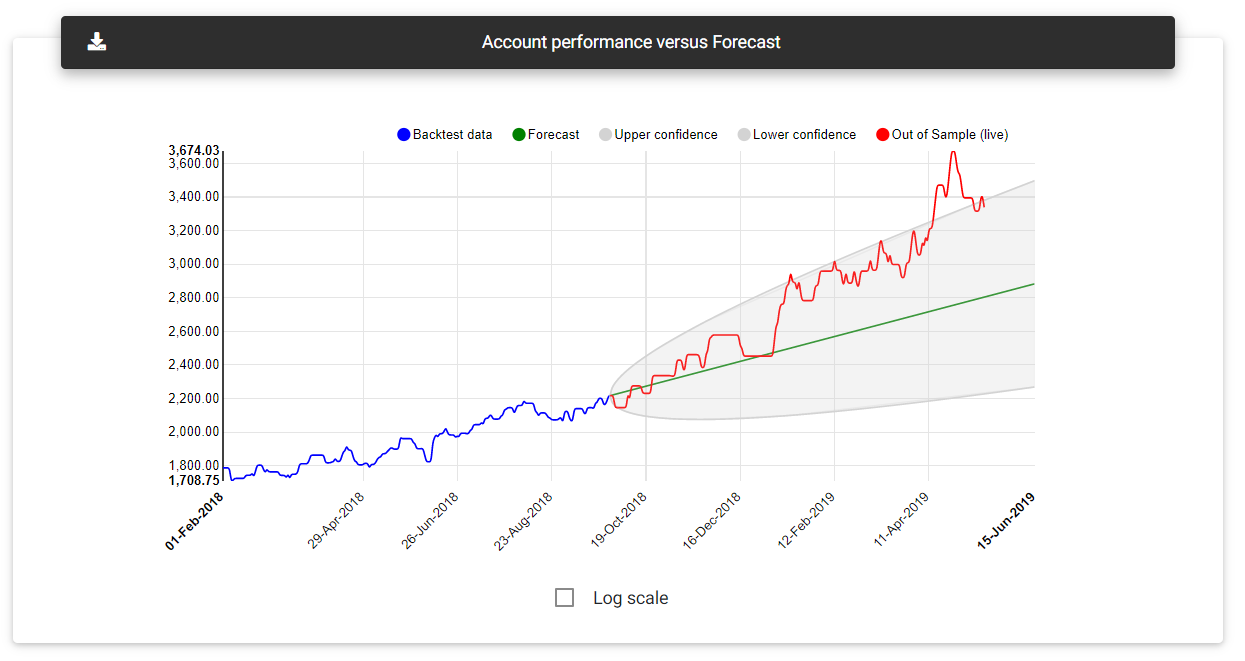Equity forecast